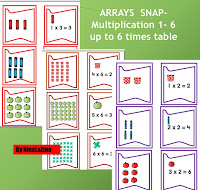# Fun ways to teach times tables Year 2 Times Tables Grade 1 Times Tables Worksheet Snap Game Colourful Maths Game Arrays

Want More Printable Maths Games - Visit My Store

I have found that by teaching children repeated addition you can introduce the concept of multiplication very  easy, quickly and in a fun way.  You can also use what is know as arrays.

If you would like to see some more fun and exciting bespoke games and worksheet, please visit my Etsy store. You will find numerous times tables activities to keep children amused for a good time

Arrays are used to get a better understanding of multiplication for year 2. The number of rows in an array represents the number of groups. The columns represent the number of items in each group.
The multiplication sentence is the number of rows times the number of columns. Children can skip count to get the answer.

3 rows  x  2 columns = 6

3 rows ( groups) x 2 columns (items in each group) = 6 total

Teaching the times tables  multiplication can be done in any order unlike division and subtraction. It is a good idea to turn them around and show how numbers could be reversed.

Kids are expected to know their multiplication tables by the year 5. There is an easy way to learn the times tables. Times tables can sometimes seem tedious and boring for children, but keep at it and do not give up. Try different methods, maths games, times tables games, math snap, online maths times tables games, rote method, repeating, learning via a song.

When children are in KS1 they learn to count in  2, 5, and 10. They can do this using a 100 square or a number line. As soon as children can count in sequence to 100 they should be taught 2, 5, 10. This is a fundamental part of them developing multiplication skills. They can do this from year 1.Children normally learn the 3 (three) times tables,  4 (four) times tables and  8 (eight) times tables in school year 3.

Children can learn the multiplication tables from a young age. Just select activities that they will enjoy!